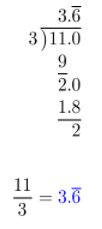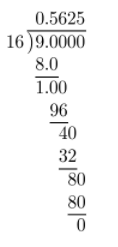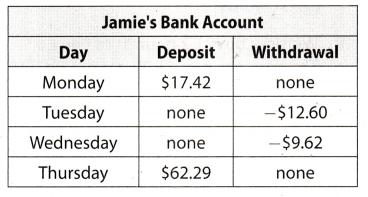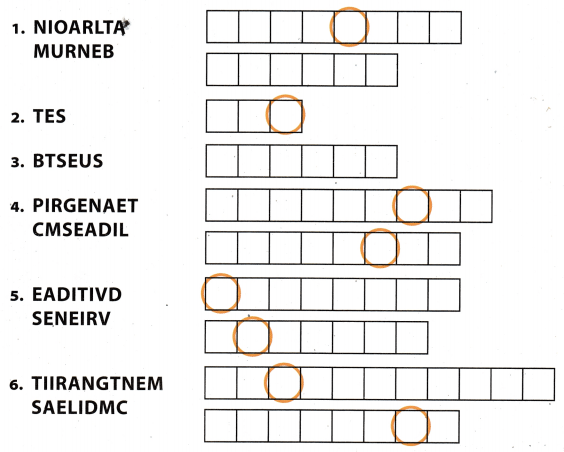Refer to our Texas Go Math Grade 7 Answer Key Pdf to score good marks in the exams. Test yourself by practicing the problems from Texas Go Math Grade 7 Unit 1 Study Guide Review Answer Key.

Write each mixed number as a whole number or decimal. Classify each number by naming the set or sets to which it belongs: rational numbers, integers, or whole numbers. (Lessons 1.1, 1.2)

Question 1.
$$\frac{3}{4}$$ _____________0.75 belongs to the set of rational numbers.

Question 2.
$$\frac{8}{2}$$ ______________4 belongs to the set of integers. the set of whole numbers and the set of rational numbers.

Question 3.
$$\frac{11}{3}$$ _______________$$3 . \overline{6}$$ belongs to the set of rational numbers.

Question 4.
$$\frac{5}{2}$$ _______________2.5 belongs to the set of rational numbers.

Find each sum or difference. (Lessons 1.3, 1.4)

Question 5.
-5 + 9.5 ____________
= 9.5 – 5
= 4.5

Question 6.
$$\frac{1}{6}$$ + (-$$\frac{5}{6}$$) ____________
= $$\frac{1}{6}$$ – $$\frac{5}{6}$$
= –$$\frac{4}{6}$$
= –$$\frac{2}{3}$$

Question 7.
-0.5 + (-8.5) _______________
= -0.5 – 8.5
= -9

Question 8.
-3 – (-8) ___________
= -3 + 8
= 5

Question 9.
5.6 – (-3.1) _________
= 5.6 + 3.1
= 8.7

Question 10.
3$$\frac{1}{2}$$ – 2$$\frac{1}{4}$$ _____________
Write mixed fractions as proper, then find a common denominator.
= $$\frac{7}{2}$$ – $$\frac{9}{4}$$
= $$\frac{14-9}{4}$$
= $$\frac{5}{4}$$

Find each product or quotient. (Lessons 1.5, 1.6)

Question 11.
-9 × (-5) __________
Product will be positive because signs are the same.
= 9 × 5
= 45

Question 12.
0 × (-7) ____________
Any number multiplied by 0 is equal to 0.
= 0 × (-7)
= 0

Question 13.
-8 × 8 _____________
The product will be negative because signs are different
= -(8 × 8)
= -64

Question 14.
– $$\frac{56}{8}$$ _______________
The quotient will be negative, because signs are different
= –$$\frac{56}{8}$$
= -7

Question 15.
$$\frac{-130}{-5}$$ _____________
The quotient will be positive, because signs are same.
= $$\frac{130}{5}$$
= 26

Question 16.
$$\frac{34.5}{1.5}$$ ______________
Write decimal numbers as fractions:
$$\frac{\frac{345}{10}}{\frac{15}{10}}$$
Write complex fraction using division:
$$\frac{345}{10}$$ ÷ $$\frac{15}{10}$$
Write using multiplication:
$$\frac{345}{10}$$ × $$\frac{10}{15}$$ = 23

Question 17.
–$$\frac{2}{5}$$ (-$$\frac{1}{2}$$) (-$$\frac{5}{6}$$) ______________
Find the product of first 2 factors. Both are negative, so the product is positive.
$$\frac{2}{5}$$ ($$\frac{1}{2}$$) = $$\frac{1}{5}$$
Multiply the result by the third factor. One is negative, one is positive, so the product is negative.
–$$\frac{1}{5}$$($$\frac{5}{6}$$) = –$$\frac{1}{6}$$

Question 18.
($$\frac{1}{5}$$) (-$$\frac{5}{7}$$) ($$\frac{3}{4}$$) _____________
Find the product of first 2 factors. One is negative, the other positive, so the product is negative.
–$$\frac{1}{5}$$ ($$\frac{5}{7}$$) = –$$\frac{1}{7}$$
Multiply the result by the third factor. One is negative, one is positive, so the product is negative.
–$$\frac{1}{7}$$ ($$\frac{3}{4}$$) = –$$\frac{3}{28}$$

Question 19.
Lei withdrew $50 from her bank account every day for a week. What was the change in her account in that week? Answer: Use negative number to represent withdrawal. Find 7 × (-50): 7 × (-50) = -350 The change on Lei’s account that week was -$350.

Question 20.
In 5 minutes, a seal descended 24 feet. What was the average rate of change in the seal’s elevation per minute?
Use negative number to represent descent in feet.
Find $$\frac{-24}{5}$$:
$$\frac{-24}{5}$$ = -4.8
Sears change in elevation is -4.8 feet per minute.

Question 1.
CAREERS IN MATH Urban Planner Armand is an urban planner, and he has proposed a site for a new town library. The site is between City Hall and the post office on Main Street.The distance between City Hall and the post office is 6$$\frac{1}{2}$$ miles. The library site is 1$$\frac{1}{4}$$ miles closer to City Hall than it is to the post office.

a. Write 6$$\frac{1}{2}$$ miles and 1$$\frac{1}{4}$$ miles as decimals.
First, write $$\frac{1}{2}$$ and $$\frac{1}{4}$$ as decimals.Then, add 6 and 1 to the result respectively.
6$$\frac{1}{2}$$ = 6 + 0.5 = 6.5 miles
1$$\frac{1}{4}$$ = 1 + 0.25 = 1.25 miles

b. Let d represent the distance from City Hall to the library site. Write an expression for the distance from the library site to the post office.
d* = distance from Library site to the Post Office
d* = d + 1.25

c. Write an equation that represents the following statement: The distance from City Hall to the library site plus the distance from the library site to the post office is equal to the distance from City Hall to the post office.
d + d* = 6.5

d. Solve your equation from part c to determine the distance from City Hall to the library site, and the distance from the post office to the library site.
d + d* = 6.5
d + d + 1.25 = 6.5
2d = 5.25
d = 2.625

d* = d + 1.25
d* = 2.625 + 1.25
d* = 3.875

Question 2.
Sumaya is reading a book with 240 pages. She has already read 90 pages. She plans to read 20 more pages each day until she finishes the book.
a. Sumaya writes the equation 330 = -20d to find the number of days she will need to finish the book. Identify the errors that Sumaya made.
First, Sumaya added 90 to 240 instead of subtracting 90 from 240. This is a mistake because if she read 90 pages, that means she has 90 pages Less to read, not more.
Second mistake is the negative sign. If the equation results in how many days more she has to read, it can not be negative.

b. Write and solve an equation to determine how many days Sumaya will need to finish the book. In your answer, count part of a day as a full day.
First, find out how many more pages she has to read:
240 – 90 = 150
Correct equation:
20d = 150
d = $$\frac{150}{20}$$
d = $$\frac{15}{2}$$
d = 7$$\frac{1}{2}$$
Sumaya will need 8 days to finish the book.

c. Estimate how many days you would need to read a book about the same length as Sumaya’s book. What information did you use to find the estimate?
Let the book have the same number of pages. I would, for example, read 10 pages a day.
10d = 330
d = 33
It would take me 33 days to read the book.

Question 3.
Jackson works as a veterinary technician and earns $12.20 per hour. a. Jackson normally works 40 hours a week. In a normal week, what is his total pay before taxes and other deductions? Answer: Find 40 × 12.20: 40 × 12.20 = 488 His total pay is$488.

b. Last week, Jackson was ill and missed some work. His total pay before deductions was $372.10. Write and solve an equation to find the number of hours Jackson worked. Answer: Find 372.10 ÷ 12.20 372.10 ÷ 12.20 = 30.5 Jackson worked 30.5 hours last week. c. Jackson records his hours each day on a time sheet. Last week when he was ill, his time sheet was incomplete. How many hours are missing? Show your work.Answer: Find 40 – 30.5 40 – 30.5 = 9.5 Jackson missed 9.5 hours last week. d. When Jackson works more than 40 hours in a week, he earns 1.5 times his normal hourly rate for each of the extra hours. Jackson worked 43 hours one week. What was his total pay before deductions? Justify your answer. Answer: His 40 hours pay is 488, as calculated iii a. He worked 43 – 40 = 3 hours overtime. Find 3 × 12.20 × 1.5: 3 × 12.20 × 1.5 = 36.6 × 1.5 = 54.9 Now add 54.9 to his 10 hours pay. Find 488 + 54.9: 488 + 54.9 = 5-12.9 Jackson’s pay that week was$542.9.

e. What is a reasonable range for Jackson’s expected yearly pay before deductions? Describe any assumptions you made in finding your answer.
Let’s say that Jackson will be sick couple of days in a year, and he will work overtime couple of days in a year. When it all adds up, assumption is he will work 40 hours a week on average. There are 52 weeks in a year.
Find 488 × 52:
488 × 52 = 25376
Jackson will probably earn somewhere in between $25000 –$26000.

Texas Go Math Grade 7 Unit 1 Mixed Review Texas Test Prep Answer Key

Selected Response

Question 1.
What is -6$$\frac{9}{16}$$ written as a decimal?
A. -6.625
B. -6.5625
C. -6.4375
D. -6.125
B. -6.5625

First, write $$\frac{9}{16}$$ as a decimal.Then, add 6 to the result.
6 + 0.5625 = 6.5625
Now, since the starting number was negative, this one has to be negative too.
-6$$\frac{9}{16}$$ = -6.5625

Question 2.
Working together, 6 friends pick 14$$\frac{2}{5}$$ pounds of pecans at a pecan farm. They divide the pecans equally among themselves. How many pounds does each friend get?
A. 20$$\frac{2}{5}$$ pounds
B. 8$$\frac{2}{5}$$ pounds
C. 4$$\frac{3}{5}$$ pounds
D. 2$$\frac{2}{5}$$ pounds
D. 2$$\frac{2}{5}$$ pounds

Start with dividing 14$$\frac{2}{5}$$ by 6:
14$$\frac{2}{5}$$ ÷ 6
Write mixed fraction as proper fraction:
$$\frac{72}{5}$$ ÷ 6
Write using multiplication:
$$\frac{72}{5}$$ × $$\frac{1}{6}$$ = $$\frac{12}{5}$$
= 2$$\frac{2}{5}$$
Each friend gets 2$$\frac{2}{5}$$ pounds.

Question 3.
What is the value of (-3.25)(-1.56)?
A. -5.85
B. -5.07
C. 5.07
D. 5.85
C. 5.07

The product will, be positive, because both factors are negative:
= 3.25(1.56)
= 5.07

Question 4.
Ruby ate $$\frac{1}{3}$$ of a pizza, and Angie ate $$\frac{1}{5}$$ of the pizza. How much of the pizza did they eat in all?
A. $$\frac{1}{15}$$ of the pizza
B. $$\frac{1}{8}$$ of the pizza
C. $$\frac{3}{8}$$ of the pizza
D. $$\frac{8}{15}$$ of the pizza
D. $$\frac{8}{15}$$ of the pizza

We have to add how much Ruby ate, and how much Angie ate
$$\frac{1}{3}$$ + $$\frac{1}{5}$$ = $$\frac{5+3}{15}$$
= $$\frac{8}{15}$$
Ruby and Angie ate $$\frac{8}{15}$$ of the pizza.

Question 5.
Jaime had $37 in his bank account on Sunday. The table shows his account activity for the next four days. What was the balance in Jaime’s account after his deposit on Thursday?A.$57.49
B. $59.65 C.$94.49
D. $138.93 Answer: C.$94.49

Use positive numbers to represent deposit. and negative numbers to represent withdrawal. Then, add it up to accounts balance before any deposits or withdrawals. 37.
37 + 17.42 – 12.60 – 9.62 + 62.29 = 54.42 – 12.60 – 9.62 + 62.29
= 41.82 – 9.62 + 62.29
= 32.2 + 62.29
= 94.49
The balance in Jaimes’s account on Friday was $94.49. Question 6. A used motorcycle is on sale for$3,600. Erik makes an offer equal to of this price. How much does Erik offer for the motorcycle?
A. $4,800 B.$2,700
C. $2,400 D.$900
B. $2,700 Start by multiplying 3600 and $$\frac{3}{4}$$: 3600 × $$\frac{3}{4}$$ = 2700 Erik offers$2700.

Question 7.
To which set or sets does the number -18 belong?
A. integers only
B. rational numbers only
C. integers and rational numbers only
D. whole numbers, integers, and rational numbers
C. integers and rational numbers only

We can see that -18 does not belong in the set of whole numbers
Next, notice that -18 belongs to the set of integers. That implies it belongs in the set of rational numbers, since the set of integers is the subset of the set of rational numbers.

Question 8.
Mrs. Rodriguez is going to use 6$$\frac{1}{3}$$ yards of material to make two dresses. The larger dress requires 3$$\frac{2}{3}$$ yards of material. How much material will Mrs. Rodriguez have left to use on the smaller dress?
A. 1$$\frac{2}{3}$$ yards
B. 2$$\frac{1}{3}$$ yards
C. 2$$\frac{2}{3}$$ yards
D. 3$$\frac{1}{3}$$ yards
C. 2$$\frac{2}{3}$$ yards

Start by subtracting 3$$\frac{2}{3}$$ = $$\frac{11}{3}$$ from 6$$\frac{1}{3}$$ = $$\frac{19}{3}$$
$$\frac{19}{3}$$ – $$\frac{11}{3}$$ = $$\frac{8}{3}$$
= 2$$\frac{2}{3}$$
Mrs. Rodriguez will have 2$$\frac{2}{3}$$ yards of material to use on the smaller dress.

Question 9.
Winslow buys 1.2 pounds of bananas. The bananas cost $1.29 per pound. To the nearest cent, how much does Winslow pay for the bananas? A.$1.08
B. $1.20 C.$1.55
D. $2.49 Answer: C.$1.55

Start by multiplying 1.2 by 1.29:
1.2 × 1.29 = 1.548
≈ 1.55
Vins1ow pays $1.55 for the bananas. Gridded Response Question 10. Roberta earns$7.65 per hour. How many hours does Roberta need to work to earn $24.48?Answer: Given earning per hour =$7.65
Given total earning of Robert = $24.48Hence, for the earning of$24.48 he need to work for 3.2 hours
The table will be made as per below instructions:
1st coLumn: mark * sign
2nd coLumn : mark 0
3rd coLumn: mark 0
4th column : mark 0
5th coLumn : mark 3
6th coLumn: mark 2
7th column: mark 0

Question 11.
What is the product of the following expression?
(-2.2)(1 .5)(-4.2)Given expression in problem: (-2.2)(1.5)(-4.2)
(-2.2)(1.5)(-4.2) = (-3.3)( 4.2)
= 13.86
The table will be made as per below instructions:
1st column: mark * sign
2nd column : mark 0
3rd column: mark 0
4th column : mark 1
5th column : mark 3
6th column: mark 8
7th column: mark 6

Hot Tip! Correct answers in gridded problems can be positive or negative. Enter the negative skin in the first column when it is appropriate. Check your work!

Question 12.
Victor is ordering pizzas for a party. He would like to have $$\frac{1}{4}$$ of a pizza for each guest. He can only order whole pizzas, not part of a pizza. If he expects 27 guests, how many pizzas should he order?Portion of pizza for each guest = $$\frac{1}{4}$$
Total number of guest in party = 27
Number of guest in 1 pizza = $$\frac{1}{\frac{1}{4}}$$ = 4
Required pizza for party = $$\frac{27}{4}$$ = 6.75
But it is mentioned in question that we can onLy order whole pizza.The required amount of pizza for 27 guest is 6.75, So Victor will order 7 pizzas.
The table will be made as per below instructions:
1st coLumn: mark – sign
2nd column : mark 0
3rd column: mark 0
4th column : mark 0
5th column : mark 7
6th column: mark 0
7th column: mark 0

Use the puzzle to preview key vocabulary from this unit. Unscramble the circled letters within found words to answer the riddle at the bottom of the page.1. Any number that can be written as a ratio of two integers. (Lesson 1-1)
2. A group of items. (Lesson 1-2)
3. A set that is contained within another set. (Lesson 1-2)
4. Decimals in which one or more digits repeat infinitely. (Lesson 1-1)
5. The opposite of any number. (Lesson 13)
6. Decimals that have a finite number of digits. (Lesson 1-1)

Question 1.
Why were the two fractions able to settle their differences peacefully?